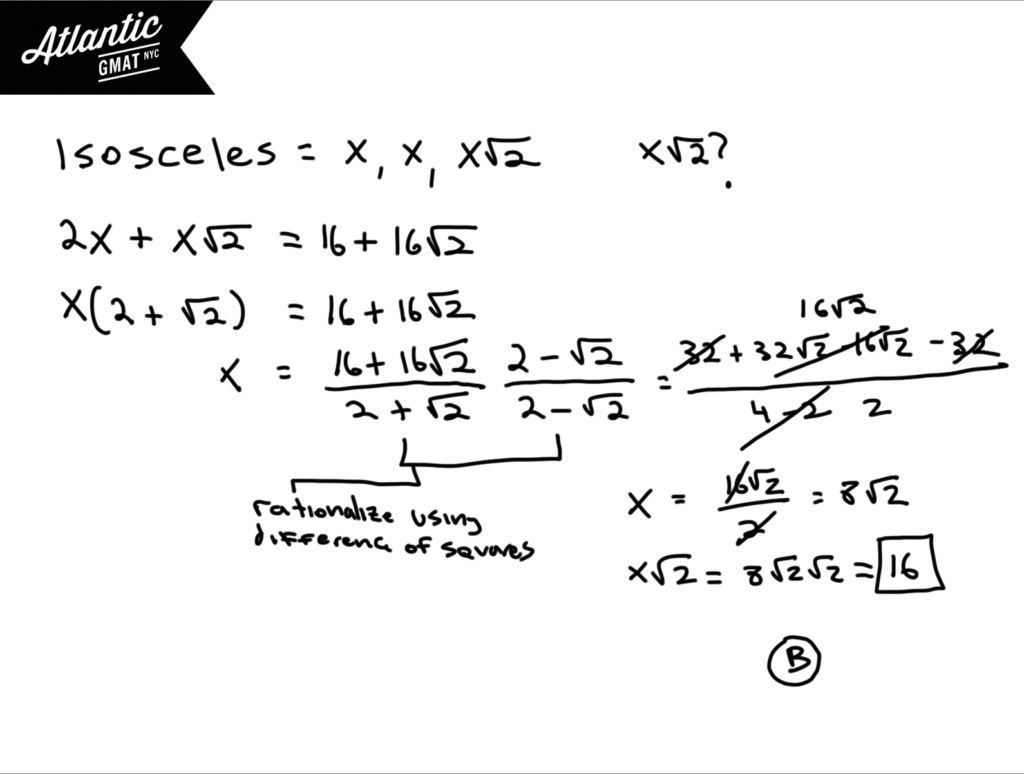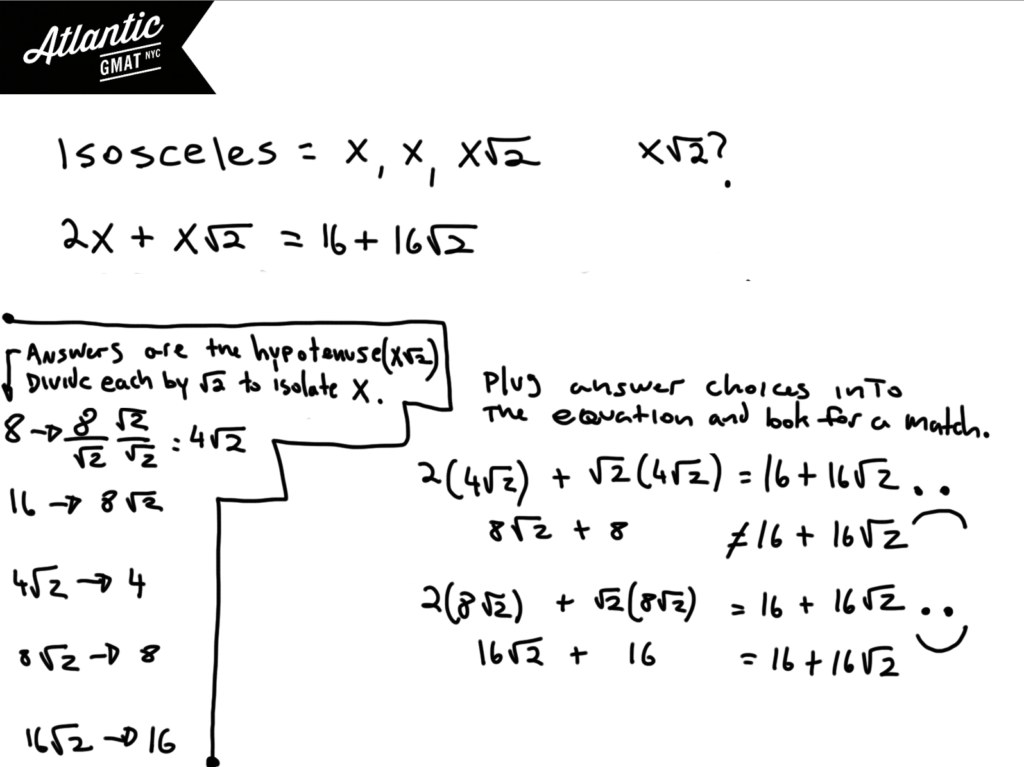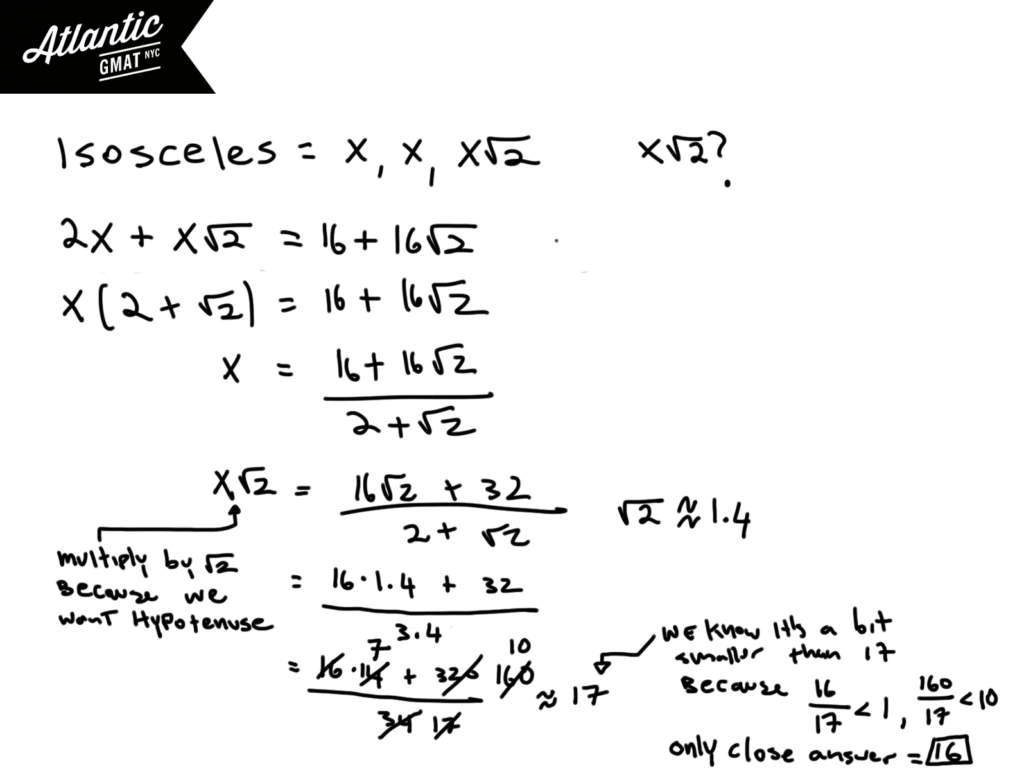Private GMAT Tutoring in NYC & Online

The perimeter of a certain isosceles right triangle is 16 + 16√2. What is the length of the hypotenuse of the triangle?

(A) 8

(B) 16

(C) 4√2

(D) 8√2

(E) 16√2

A lot of people get this GMAT geometry question from the GMAT prep software wrong. It's true that the algebra gets a little tricky but conceptually there's not much going on. And, to add, there are three ways to solve this thing. You can tackle the algebra, plug in answer choices, or you can even estimate. We're going to take a look at each solution. Let's start with the algebraic one. You need to know that the sides of an isosceles right triangle are always in the ratio x, x, x√2. No excuses for not knowing GMAT basics. You have to be fluent with the fundamentals so that you can focus on what the test is really about: critical thinking. Back to the solution! The perimeter then is 2x + x√2. And that equals 16 + 16√2. About half of people stop here and stay: Bingo! The hypotenuse must be 16√2!!! Nope. That's some fuzzy logic. Let's keep simplifying by trying to isolate the variable. You can do that by factoring out the x. Do you know how to get rid of radicals in the denominator (rationalizing)? Well, that's another basic that you'll need to conquer the GMAT quant. Keep in mind that we're solving for x√2 (the hypotenuse). See the diagram below for the worked out solution and/or check out the video solution here: the perimeter of a certain isosceles right triangle is 16You can also use the answer choices. I'd still do a bit of simplifying but then you can plug in the answers and see if the equation stays balanced (what is on the left equals what is on the right). Again, keep in mind that the answers are the hypotenuse, x√2, not x. So in order to plug them in for x we need to divide them by √2. Sounds more complicated than it is. Plugging in answers ends up being even easier than the algebra.Last but not least you can estimate. Estimation is a very helpful tool for getting through some GMAT questions. My advice is:

1. Keep your approximation/estimation in line with how far apart the answer choices are. In this case our approximations are within 10% and the closest answers are about 30% apart giving us some breathing room.
2. Use estimation/approximation as a useful tool deployed when appropriate not as a crutch for when you're in panic mode.

Here we just have to call √2 1.4. After that the arithmetic is basic. You can multiply everything by 10 to get rid of the decimals and then simplify. 16/17 is so close to 1 that we can just call it 1. 160/17 is very close to 10. With that we get 17. But we know that we're a tiny bit less than 17. The only answer that's remotely close is 16. 16√2 is almost 24. 8√2 is little less than 12. Those are way too far off to be considered. Comment with any questions or addition. Happy studies!# CONTACT

Atlantic GMAT

405 East 51st St.

NY, NY 10022

(347) 669-3545

info@AtlanticGMAT.com